Home > CCA2 > Chapter 12 > Lesson 12.2.3 > Problem12-136

12-136.
1.Without using a calculator, solve these two problems from a college entrance exam. Homework Help ✎

1. If x−4/3 + 1 = 17, find x−1/3.

2. If log(A) = a, find log(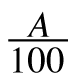).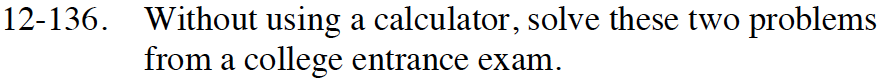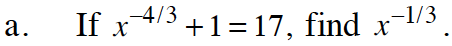Solve the first equation for x, then use that value to find the answer.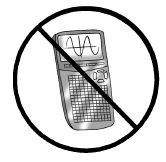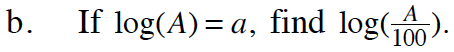a − 2